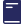Over 1000 Titles for students & teachers

# Excel Basic Skills - Working With Numbers Year 2

\$14.95

Excel Basic Skills: Working With Numbers Year 2 contains over 100 activities on number skills such as sequencing, adding, trading, subtracting, multiplying, dividing and working with fractions. The range of interesting, graded questions will help Year2 students become confident in working with numbers.

In this book you will find:

• a wide variety of interesting exercises
• words associated with each topic highlighted to ensure understanding
• two review tests to assess your child's progress
This book has been written to develop basic skills in number work for children in their third year of school (Year 2 in NSW). It is assumed that writing and reading skills have been developing over the last two years. Children should have worked with numbers 1-99 and been introduced to elementary addition and subtraction.

Contents:

• recognising numerals 0 to 99
• sequencing numbers 0 to 99
• introducing the number 100
• recognising numerals 0 to 999
• sequencing numbers 0 to 999
• introducing fractions
• review
• beginning subtraction
• subtracting to 20
• introducing multiplication
• multiplying by 2
• introducing division by sharing
• introducing division by grouping
• review

#### Product Details

SKU:
9781864413397
YEAR:
Year 2
Publication Edition Date:
2004/10/01
Dimensions:
270 mm (h) x 210 mm (w)
ISBN:
9781864413397
BRAND:
Excel
SUB BRAND:
Excel Basic Skills
SUBJECT:
Mathematics
Format:
Paperback
Author(s):